TitleCollege Algebra
Tutorial 20:
Equations that are Quadratic in Form

WTAMU > Virtual Math Lab > College AlgebraLearning Objectives

 After completing this tutorial, you should be able to: Solve equations that are quadratic in form.IntroductionTutorial

 Quadratic in Form An equation is quadratic in form when it can be written in this standard form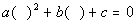where the same expression is inside both (  )'s.

 In other words, if you have a times the square of the expression following b plus b times that same expression not squared plus c equal to 0, you have an equation that is quadratic in form. If we substitute what is in the (   ) with a variable like t, then the original equation will become a quadratic equation.

 Solving  Equations that are  Quadratic in Form

Step 1:  Write in Standard Form,, if needed.

 If it is not in standard form, move any term(s) to the appropriate side by using the addition/subtraction property of equality.  Also, make sure that the squared term is written first left to right, the expression not squared is second and the constant is third and it is set equal to 0.

Step 2: Substitute a variable in for the expression that follows b in the second term.

 In other words, substitute your variable for what is in the (   ) when it is in standard form,. I’m going to use t for my substitution, but really you can use any variable as long as it is not the variable that is used in the original equation.

Step 3: Solve the quadratic equation created in step 2.

 If you need a review on solving quadratic equations, feel free to go to Tutorial 17:  Quadratic Equations. You can use any method you want to solve the quadratic equation: factoring, completing the square or quadratic formula.

Step 4: Find the value of the variable from the original equation.

 Keep in mind that you are finding a solution to the original equation and that the variable you substituted in for in step 2 is not your original variable.  Use the substitution that was used to set up step 2 and then solve for the original variable.

 In some cases, you will be working with rational exponents and square roots in your problems.  Those types of equations can cause extraneous solutions.  Recall that an extraneous solution is one that is a solution to an equation after doing something like raising both sides of an equation by an even power, but is not a solution to the original problem. Even though not all of the quadratic in form equations can cause extraneous solutions, it is better to be safe than sorry and just check them all.Example 1: Solve the equation that is quadratic in form: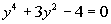.

 Step 1:  Write in Standard Form,, if needed.

 This equation is already in standard form.

 Below, I have the original equation rewritten in a way to show you that it is quadratic in form.  Note how when you square y squared you get y to the fourth, which is what you have in the first term.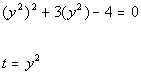*Rewriting original equation to show it is quadratic in form *Note that (y squared) squared = y to the fourth *When in stand. form, let t = the expression following b.

 Next, we need to substitute t in for y squared in the original equation.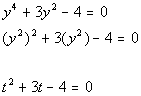*Original equation     *Substitute t in for y squared

 Note how we ended up with a quadratic equation when we did our substitution.  From here, we need to solve the quadratic equation that we have created.

 Step 3: Solve the quadratic equation created in step 2.

 You can use any method you want to solve the quadratic equation: factoring, completing the square or quadratic formula. I'm going to factor it to solve it.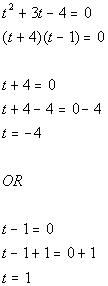*Use Zero-Product Principle *Set 1st factor = 0 and solve           *Set 2nd factor = 0 and solve

 In step 2 we used the substitution of t = y squared.

 Let's find the value(s) of y when t = -4: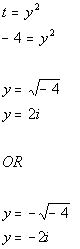*Plug in - 4 for t *Use square root method to solve for y *First solution         *Second solution

 Let's find the value(s) of y when t = 1: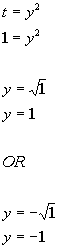*Plug in 1 for t *Use square root method to solve for y *First solution         *Second solution

 Let's double check to see if y = 2i is a solution to the original equation.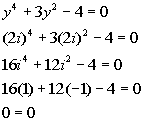*Plugging in 2i for y *i squared simplifies to be -1 *i to the fourth simplifies to be 1 *True statement

 Since we got a true statement, y = 2i  is a solution.

 Let's double check to see if y = -2i is a solution to the original equation.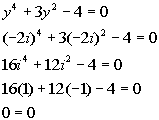*Plugging in -2i for y *i squared simplifies to be -1 *i to the fourth simplifies to be 1 *True statement

 Since we got a true statement, y = -2i  is a solution.

 Let's double check to see if y = 1 is a solution to the original equation.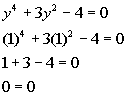*Plugging in 1 for y *True statement

 Since we got a true statement, y = 1  is a solution.

 Let's double check to see if y = -1 is a solution to the original equation.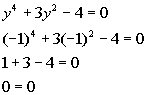*Plugging in -1 for y *True statement

 Since we got a true statement, y = -1  is a solution.

 There are four solutions to this equation: y = 2i, y = -2i, y = 1, and y = -1.Example 2: Solve the equation that is quadratic in form: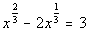.

 Step 1:  Write in Standard Form,, if needed.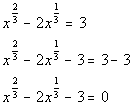*Inverse of add. 3 is sub. 3 *Equation in standard form

 Below, I have the original equation rewritten in a way to show you that it is quadratic in form.  Note how when you square x to the 1/3 power you get x to the 2/3 power, which is what you have in the first term.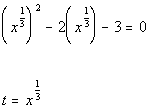*Rewriting original equation to show it is quadratic in form *Note that (x to the 1/3 power) squared = x to the 2/3 power *When in stand. form, let t = the expression following b.

 Next, we need to substitute t in for x to the 1/3 power in the original equation.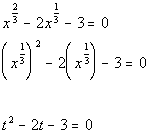*Original equation     *Substitute t in for x to the 1/3 power

 Note how we ended up with a quadratic equation when we did our substitution.  From here, we need to solve the quadratic equation that we have created.

 Step 3: Solve the quadratic equation created in step 2.

 You can use any method you want to solve the quadratic equation: factoring, completing the square or quadratic formula. I'm going to factor it to solve it.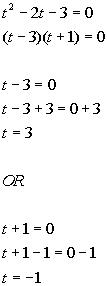*Use Zero-Product Principle *Set 1st factor = 0 and solve           *Set 2nd factor = 0 and solve

 In step 2 we used the substitution of t = x to the 1/3 power.

 Let's find the value(s) of x when t = 3: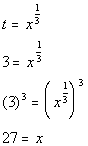*Plug in 3 for t *Solve the rational exponent equation *Inverse of taking it to the 1/3 power is raising it to the 3rd power

 Let's find the value(s) of x when t = -1: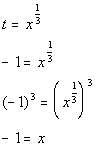*Plug in -1 for t *Solve the rational exponent equation *Inverse of taking it to the 1/3 power is raising it to the 3rd power

 Let's double check to see if x = 27 is a solution to the original equation.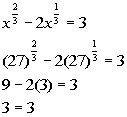*Plugging in 27 for x   *True statement

 Since we got a true statement, x = 27  is a solution.

 Let's double check to see if x = -1 is a solution to the original equation.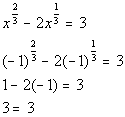*Plugging in -1 for x   *True statement

 Since we got a true statement, x = -1  is a solution.

 There are two solutions to this equation: x = 27 and x = -1.Example 3: Solve the equation that is quadratic in form: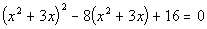.

 Step 1:  Write in Standard Form,, if needed.

 This equation is already in standard form.

 Note how the original equation has the exact same expression in the two (  )'s and that the first (  ) is squared and the 2nd (  ) is to the one power.  This equation is quadratic in form.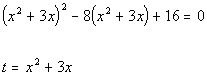*When in stand. form, let t = the expression following b.

 Next, we need to substitute t in for x squared plus 3x in the original equation.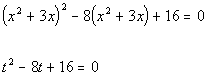*Original equation   *Substitute t in for x squared plus 3x

 Note how we ended up with a quadratic equation when we did our substitution.  From here, we need to solve the quadratic equation that we have created.

 Step 3: Solve the quadratic equation created in step 2.

 You can use any method you want to solve the quadratic equation: factoring, completing the square or quadratic formula. I'm going to factor it to solve it.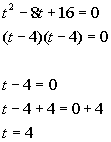*Use Zero-Product Principle *Set 1st factor = 0 and solve *2nd factor is the same as the 1st

 In step 2 we used the substitution of t = x squared plus 3x.

 Let's find the value(s) of x when t = 4: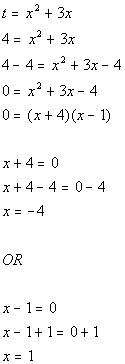*Plug in 4 for t *Solve the quadratic eq. for x *Factor the trinomial   *Use Zero-Product Principle *Set 1st factor = 0 and solve *First solution       *Set 2nd factor = 0 and solve   *Second solution

 Let's double check to see if x = -4 is a solution to the original equation.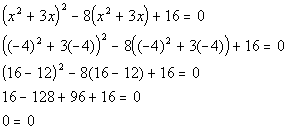*Plugging in -4 for x     *True statement

 Since we got a true statement, x = -4  is a solution.

 Let's double check to see if x = 1 is a solution to the original equation.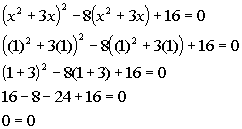*Plugging in 1 for x       *True statement

 Since we got a true statement, x = 1  is a solution.

 There are two solutions to this equation: x = -4 and x = 1.Practice Problems

 These are practice problems to help bring you to the next level.  It will allow you to check and see if you have an understanding of these types of problems. Math works just like anything else, if you want to get good at it, then you need to practice it.  Even the best athletes and musicians had help along the way and lots of practice, practice, practice, to get good at their sport or instrument.  In fact there is no such thing as too much practice. To get the most out of these, you should work the problem out on your own and then check your answer by clicking on the link for the answer/discussion for that  problem.  At the link you will find the answer as well as any steps that went into finding that answer.Practice Problems 1a - 1c: Solve each quadratic in form equation.

 1a.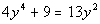(answer/discussion to 1a) 1b.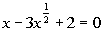(answer/discussion to 1b)

 1c.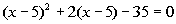(answer/discussion to 1c)Need Extra Help on these Topics?

 No appropriate web pages could be found to help you with the topics on this page.    Go to Get Help Outside the Classroom found in Tutorial 1: How to Succeed in a Math Class for some more suggestions.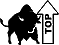WTAMU > Virtual Math Lab > College Algebra

Videos at this site were created and produced by Kim Seward and Virginia Williams Trice.
Last revised on Dec. 16, 2009 by Kim Seward.